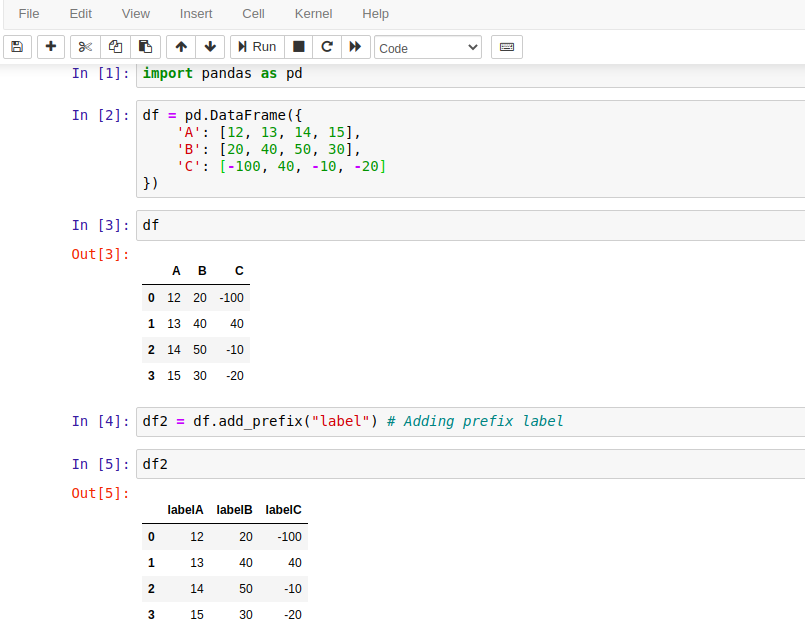# Python | Pandas DataFrame add_prefix() Method

Pandas add_prefix() method is a built-in method of DataFrame that is used to add a prefix to row label and columns label in Series and DataFrame respectively.

If we call this method on Series then it adds prefix labels with string prefix to row label similarly it adds prefix with columns if called on DataFrame. Prefix value is a string value that is concatenated with the previous label value.

For example, if a DataFrame has a column label as A then adding "Value" as a prefix will modify it as ValueA. For more details see the examples below.

## Syntax

``DataFrame.add_prefix(prefix)``

### Parameters:

prefixstr: The string to add before each label.

### Return Value:

It returns either a new Series or new DataFrame with updated labels.

## Example with Jupyter Notebook

Let's take an example to understand the use of pandas.DataFrame.add_prefix() method. Following is the code that is executed by using the Jupyter notebook. If you are familiar with Jupyter, See the screenshot below.## Example: How to Add Column Label to a DataFrame in Pandas?

We can use DataFrame.add_prefix() method to add string prefix for column labels in DataFrame. See the example here. This example is similar to the above code, executed by using Jupyter notebook. If you are not familiar with Jupyter then see this code to understand.

``````# import pandas library
import pandas as pd
# Create DataFrame of data
df = pd.DataFrame({
'A': [12, 13, 14, 15],
'B': [20, 40, 50, 30],
'C': [-100, 40, -10, 20]
})
# print dataframe
print(df)
print("\nDataFrame with updated labels...\n")
print(df2)
``````

Output:

A   B    C
0
12  20 -100
1  13  40   40
2  14  50  -10
3  15  30   20

DataFrame with updated labels...

labelA  labelB  labelC
0
12      20    -100
1      13      40      40
2      14      50     -10
3      15      30      20

## Example: Add Row Label To a Series in Pandas

If we are working with Series and want to modify its label by adding some prefix string then use the add_prefix() method. It returns a Series after modifying its labels. See the example here.

``````# import pandas library
import pandas as pd
# Create Series of data
sr = pd.Series([12, 13, 14, 15])
# print Series
print(sr)
print("\nSeries with updated labels...\n")
print(sr2)
``````

Output:

0    12
1    13
2    14
3    15
dtype: int64

Series with updated labels...

label0    12
label1    13
label2    14
label3    15

## Example: Create DataFrame using Numpy in Pandas

Here we have a DataFrame created by using the NumPy library and we are using the add_prefix() method to append column labels. See the example here.

``````# import pandas and numpy library
import pandas as pd
import numpy as np
# Creating DataFrame using numpy Array
df = pd.DataFrame(np.random.rand(5, 3), columns =['A', 'B', 'C'])
# Print DataFrame
print(df)
print("\nNew Dataframe after updating labels...\n")
# Print new dataframe
print(df2)
``````

Output:

A         B         C
0  0.267441  0.471324  0.876935
1  0.490577  0.622735  0.688777
2  0.171497  0.511526  0.813753
3  0.970845  0.249137  0.839091
4  0.667099  0.313956  0.486564

New Dataframe after updating labels...

RandomValuesA  RandomValuesB  RandomValuesC
0       0.267441       0.471324       0.876935
1       0.490577       0.622735       0.688777
2       0.171497       0.511526       0.813753
3       0.970845       0.249137       0.839091
4       0.667099       0.313956       0.486564

Trending